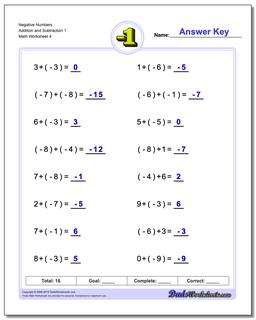# Math Worksheets: Negative Numbers: Negative Numbers: Negative Numbers Addition and Subtraction 1 (Fourth Worksheet)## Negative Numbers Addition and Subtraction 1 (Fourth Worksheet)

PropertyValue
DescriptionNegative Numbers Addition and Subtraction 1: Worksheets for adding negative numbers and subtracting negative numbers. (Fourth Worksheet)
Resource TypeWorksheet